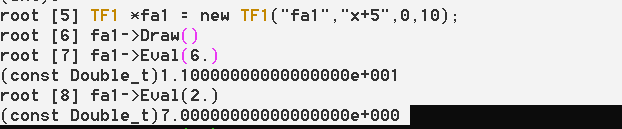# How to use Eval(...) method for a function in TF1!

Dear all,

I have long code, but I will post the small portion to make the point. Normally, I create and fill a histogram, then I fit these functions on to the histogram. In the end, I need to read a Y value for a corresponding X value from a fitted gaussian function. I don’t want to use GetBinContent(bin) method to get the result from a histogram, instead.

Note that I made up the numbers to simplify the situation.

If I create a gaussian function on a terminal instead of writing in a macro, I can call Y value for any X value with Eval(). It seems getting he value from a fitted gaussian ruins everything. I used to run it OK before.TF1 *g1 = new TF1(“g1”,“gaus”, 0, 1024 );
TF1 *pol1 = new TF1(“pol1”,“pol1”, 0, 1024 );
TF1 *total = new TF1(“total”,“gaus(0)+pol1(3)”, 0, 1024);

h1->Fit(g1,“R”);
h1->Fit(pol1,“R+”);
double par;
g1->GetParameters(&par);
pol1->GetParameters(&par);
total->SetParameters(par);
h1->Fit(total, “RNQ+”);
double p0 = par;
double p1 = par;
double p2 = par;
double p3 = par;
double p4 = par;
double e0 = g1->GetParError(0);
double e1 = g1->GetParError(1);
double e2 = g1->GetParError(2);
double e3 = pol1->GetParError(0);
double e4 = pol1->GetParError(1);
g1->Draw(“same”);
cout <<"!!!: 310. channel" << g1->Eval(310.) << " 320. channel " << g1->Eval(320.) <<endl;

This last cout line doesn’t read the Y value from a fitted function.

After the last “Fit” statement, try (see / compare the displayed values of parameters):
`g1->Print("V"); pol1->Print("V"); total->Print("V");`

BTW. It’s probably better to use:
`TF1 *pol1 = new TF1("MyPol1", "pol1", 0., 1024.);`

This option doesn’t seem doing the trick except printing the details. The problem of Eval() stays put.

Test “Eval” (note: “g1” is just a “Gaussian”):
`std::cout << g1->Eval(g1->GetParameter(1)) << " = " << g1->GetParameter(0) << std::endl;`

Your suggestion works perfectly and cleverly fine. I updated the code. Do you think the problem was the definition of the parameters which should have suited the definition of TF1 in ROOT? Probably, I set up them int before !

FindFittingValues.cpp (7.2 KB)

Co60.txt (3.6 KB)

Updated results match:

cout <<"!!!TESTING1!!!: 313.533 " << g1->Eval(313.533,0.,0.,0.) << endl;
std::cout <<"!!!TESTING2!!!: @channel " <GetParameter(1) <<", the count is: "<< g1->Eval(g1->GetParameter(1)) << " which equals to " << g1->GetParameter(0) << std::endl;

As a next step, I want to find the X and Y axis points where 2 Gaussians intercepts. What method can I try except solving the 2 Gaussian equations by making them equal to each other to find the common solution point?